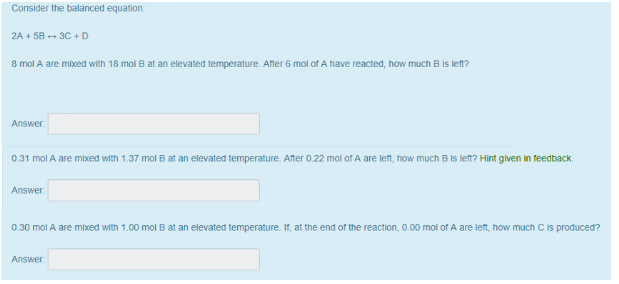# Problem: Consider the balanced equation: 2A + 5B &lt;—&gt; 3C + D (A) 8 mol A are mixed with 18 mol B at an elevated temperature. After 6 mol of A have reacted, how much B is left? (B) 0.31 mol A are mixed with 1.37 mol B at an elevated temperature. After 0.22 mol of A are left, how much B is left? (C) 0.30 mol A are mixed with 1.00 mol B at an elevated temperature. If, at the end of the reaction, 0.00 mol of A are left, how much C is produced?

###### FREE Expert Solution
92% (490 ratings)###### Problem Details

Consider the balanced equation:

2A + 5B <—> 3C + D

(A) 8 mol A are mixed with 18 mol B at an elevated temperature. After 6 mol of A have reacted, how much B is left?

(B) 0.31 mol A are mixed with 1.37 mol B at an elevated temperature. After 0.22 mol of A are left, how much B is left?

(C) 0.30 mol A are mixed with 1.00 mol B at an elevated temperature. If, at the end of the reaction, 0.00 mol of A are left, how much C is produced?